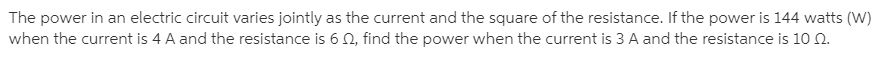# The power in an electric circuit varies jointly as the current and the square of the resistance. If the power is 144 watts (W) when the current is 4 A and the resistance is 6 Q, find the power when the current is 3 A and the resistance is 10 .

Questionhelp_outlineImage TranscriptioncloseThe power in an electric circuit varies jointly as the current and the square of the resistance. If the power is 144 watts (W) when the current is 4 A and the resistance is 6 Q, find the power when the current is 3 A and the resistance is 10 . fullscreen